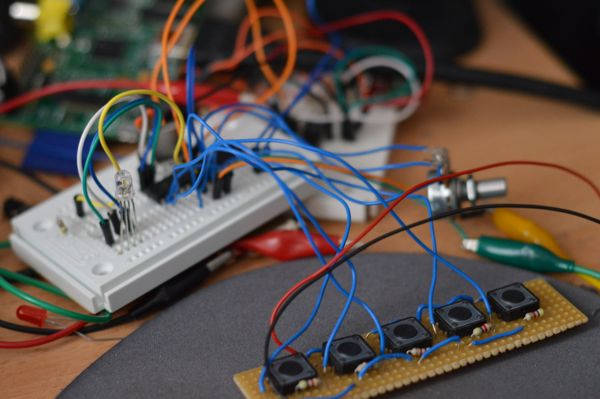## Day 26 of 30DaysOfCreativity - prototyping the controls

Today I hooked up the RGB led, the buttons and the potentionmeter to my breadboard arduino. I run the ATMega328P with a 20Mhz crystal and 3.3V which works pretty well. All my problems with I2C stability have disappeared now. The potentiometer is hooked to A0, the buttons are on D9 to D14 and the RGB led is connected to D6, D7 and D8. If the I2C master sends 1,2 or 3 the led color is changed, otherwise the slave sends the value of the potentiometer and the state of the buttons.This is the code that creates the i2C slave on the arduino side

``````#include <Wire.h>

int analog;
int buttons;
byte state;
byte data;

void setup() {
data = 0;
data = 0;
data = 0;

for ( int i=0; i<5; i++) {
pinMode( i+9, INPUT );
}

pinMode( 6, OUTPUT );
pinMode( 7, OUTPUT );
pinMode( 8, OUTPUT );

buttons = 0;
analog = 0;

digitalWrite( 6, HIGH );
digitalWrite( 7, HIGH );
digitalWrite( 8, LOW );

Wire.onRequest( requestEvent );
}

void receiveEvent( int c ) {
while ( Wire.available()) {
if ( state == 1 ) {
digitalWrite( 6, LOW );
digitalWrite( 7, HIGH );
digitalWrite( 8, HIGH );
} else if ( state == 2 ) {
digitalWrite( 6, HIGH);
digitalWrite( 7, LOW );
digitalWrite( 8, HIGH );
} else if ( state == 3) {
digitalWrite( 6, HIGH);
digitalWrite( 7, HIGH );
digitalWrite( 8, LOW );
}
}
}

void requestEvent() {
data = (byte) (analog >> 8);
data = (byte) (analog & 0xFF);
data = (byte) (buttons & 0xFF);

Wire.write( data, 3 );
buttons = 0;
}

void loop() {
delay(5);
delay(5);

analog = (a + b + c)/3;

for( int i=0; i<5; i++ ) {
if (digitalRead( i+9 ) == HIGH ) buttons |= ( 1 << i );
}
}
``````

and is a short python test program that runs on the raspberry. The code cycles through the rgb led and then reads the state of the buttons and the potentiometer

``````import smbus
import time
bus = smbus.SMBus(1)

time.sleep(1)

time.sleep(1)

time.sleep(1)

a = res
b = res
c = res

print (a * 255 + b), bin(c)
``````